# Complex projective plane

## Definition

The complex projective plane is the complex projective space of complex dimension 2. As a manifold over the reals, it has dimension 4. It is denoted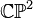$\mathbb{C}\mathbb{P}^2$ or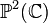$\mathbb{P}^2(\mathbb{C})$.

Alternatively, it can be viewed as the quotient of the space$S^2(\mathbb{C}) \cong S^5$ under the action of$S^0(\mathbb{C}) \cong S^1$ by multiplication. In particular, there is a fibration$S^1 \to S^5 \to \mathbb{C}\mathbb{P}^2$.

## Algebraic topology

### Homology groups

Further information: homology of complex projective space

The homology groups with coefficients in$\mathbb{Z}$ are as follows: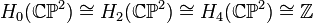$H_0(\mathbb{C}\mathbb{P}^2) \cong H_2(\mathbb{C}\mathbb{P}^2) \cong H_4(\mathbb{C}\mathbb{P}^2) \cong \mathbb{Z}$, and all other homology groups are zero.

More generally, the homology group with coefficients in a module$M$ over a commutative unital ring$R$ are as follows:$H^0(\mathbb{C}\mathbb{P}^2;M) \cong H^2(\mathbb{C}\mathbb{P}^2;M) \cong H^4(\mathbb{C}\mathbb{P}^2;M) \cong M$, and all other homology groups are zero.

### Cohomology groups

Further information: cohomology of complex projective space

The cohomology groups with coefficients in$\mathbb{Z}$ are as follows: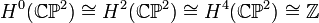$H^0(\mathbb{C}\mathbb{P}^2) \cong H^2(\mathbb{C}\mathbb{P}^2) \cong H^4(\mathbb{C}\mathbb{P}^2) \cong \mathbb{Z}$, and all other cohomology groups are zero. The cohomology ring is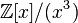$\mathbb{Z}[x]/(x^3)$ where$x$ is an additive generator for the second cohomology group.

More generally, the cohomology group with coefficients in a commutative unital ring$R$ are as follows:$H^0(\mathbb{C}\mathbb{P}^2;R) \cong H^2(\mathbb{C}\mathbb{P}^2;R) \cong H^4(\mathbb{C}\mathbb{P}^2;R) \cong R$, and all other cohomology groups are zero. The cohomology ring is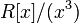$R[x]/(x^3)$ where$x$ is a$R$-module generator for the second cohomology module.

### Homotopy groups

Further information: homotopy of complex projective space

The homotopy groups are as follows:

Value of$k$ General name for homotopy group/set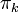$\pi_k$ What is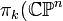$\pi_k(\mathbb{C}\mathbb{P}^n$ for generic$n \ge 2$?) What is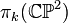$\pi_k(\mathbb{C}\mathbb{P}^2)$?
0 set of path components one-point set one-point set, so$\mathbb{C}\mathbb{P}^2$ is a path-connected space
1 fundamental group trivial group trivial group, so$\mathbb{C}\mathbb{P}^2$ is a simply connected space.
2 second homotopy group$\mathbb{Z}$$\mathbb{Z}$
3 third homotopy group trivial group trivial group
4 fourth homotopy group trivial group trivial group
5 fifth homotopy group$\mathbb{Z}$ if$n = 2$, zero otherwise$\mathbb{Z}$$k \ge 6$ Same as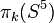$\pi_k(S^5)$